Smartick is an online platform for children to master math in only 15 minutes a day

Nov19

# How to Add 3 Fractions with Different Denominators

On the Smartick blog, we have written a lot about fractions and the different operations we can do with them. In today’s blog post you can find much more information!

Until now, we had not written about operations with more than 2 fractions.

In this post, you are going to learn to add 3 fractions with different denominators with help from a visual aid. That way, you can understand the mathematical process behind the calculation.

#### How to add 3 fractions with different denominators (two of which are multiples)

We are going to begin with the following addition problem: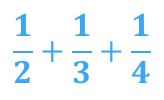To understand this problem correctly, we have graphically represented each addend: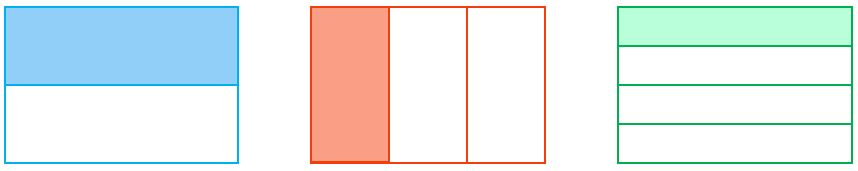Using a rectangle as the unit, we divide them into 2, 3, and 4 parts, and in this case, each is a different color. Now we have represented the 3 addends: 1/2, 1/3, and 1/4.

First, we divide each rectangle into equal parts (adding the divisions to each rectangle):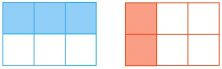Now you can solve it easily! The two are shown in ”sixths” and that way we get the following sum: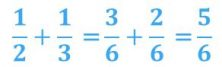We can represent the sum of this equation like this: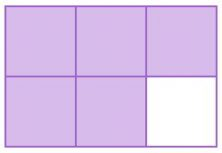Now all that’s left is to add the third addend, which we can do like this: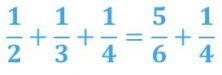And can be graphically represented like this: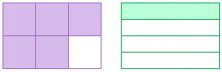To be able to add them, we divide them into equal parts like before: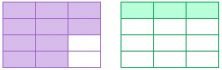We’ve converted the 2 fractions into ”twelfths” and now have the sum: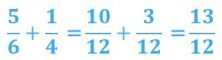That we can represent in the following way: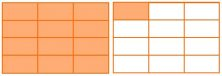Have you seen how easy it is to add fractions?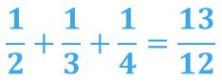Visualizing everything makes it much easier!

#### How to add 3 fractions with different prime number denominators

Now we are going to solve a problem that is a bit more difficult because the denominators are different prime numbers and we need to go through the process a bit more carefully: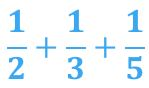But as you can see, with the visual help it makes it easier as well!

Like before, we graphically represent each fraction: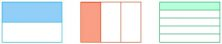Since the sum of the first two is the same as before, we have already calculated that answer above:And we have the following sum: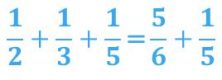For an answer that is much easier to add, we represent 5/6 as a rectangle divided vertically and 1/5  as a rectangle divided horizontally: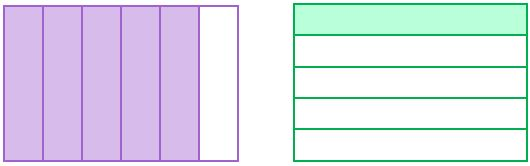We divide both representations into equal parts: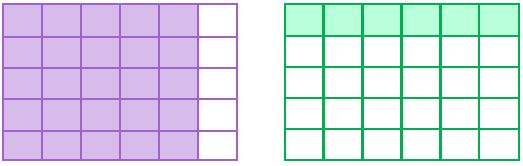Now we have the 2 fractions in ”thirtieths” and the sum: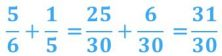That can be represented like this: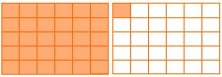You’ve solved it!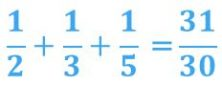I hope that it was easy to understand how to add 3 fractions. Share this post if you like it!

Latest posts by Smartick (see all)

• LeonardoSep 30 2019, 11:49 AM

Hi there,

Firstly, thank you for all the info and help that you provide.

Now, in the first 3 “image aid” the 3rd is wrongly represented. Since you are teaching and helping people, you should be more careful with your blog post review because it can confuse whoever is trying to use it as a source of learning or information. I say that because when we come to sources like this we always have in mind that everything that is being posted was carefully revised and approved what can bring us to think that we can trust these info and use them to really learn with it. And in a situation like that a great explanation like you guys provided here, can be even more confusing than helpful.

I hope you take it as a compliment for all your hard work and to always make it better.

Sincerely,